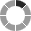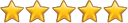# Auto Populate Fields

Need to auto populate value based on the value of related field? For this requirement Auto Populate is the perfect solution. The advanced configuration tools allow you to clearly define what should happen whenever a related field value changes.

## Introduction

Auto Populate Fields Extension allows you to Auto Populate specific fields of Source Module Using Related Field while Creating/Editing a Record.

## Features

• Auto Populate Related Module fields value in Source Module using Related Field.
• Auto Populate Fields Extension supports on Create/Editview of Module.
• Auto Populate Fields Extension support on Quick Create from Subpanel as well.
• Configure the Field Mapping & Calculate Field of all the Related Field of Source Modules using Single Configuration.
• Auto Populate value in Source Module using Related Field for Mathematical and Logical functions(Addition, Subtraction, Multiplication, Add Month, Add Year, Sub Month, Sub Year, etc).
• Active/Inactive/Delete any Auto Populate Field Configuration by one click from Listview of Auto Populate Fields Extension Configuration Page using Bulk Action Dropdown option.
• Auto Populate Fields support Multi Languages (German, English, Spanish, French, Hungarian, Italian, Dutch, Portuguese, Russian, Ukrainian)

## Functions syntax for Calculate Field

• Add : add(field1,....): Returns the sum of given numbers in field. For e.g : add(1,5,3) = 9
• Subtract : sub(field1, field2) : Returns the subtraction of given numbers in field. For e.g : sub(4,2) = 2
• Multiply : mul(field1,...) : Returns the multiplication of given numbers in field, For e.g: mul(2,3) = 6
• Divide : div(field1_numerator_field, field2_denominator_field) : Returns the field1_numerator_field divided by field2_denominator_field. For e.g: div(20,2) = 10
• String Length : strlen(Stringfield) : Returns the total length of string. For e.g : strlen('SuiteCRM') = 8
• Concatenation : concat(Stringfield,...) : Returns the string that results from concatenating the arguments. For e.g : concat(Auto, ,Populate, Fields) = Auto Populate Fields
• Log : log(numberField,base) : Returns the logarithm of a number with applied base. For e.g : log(1000,10) = 3
• Ln : ln(numberField) : Returns the natural log of a number. For e.g. : ln(10) = 2.302585
• Absolute : abs(numberField) : Returns the absolute value of a number. For e.g : abs(-24) = 24
• Average : avg(numberField,...) : Return the average of provided numbers. For e.g : avg(2,4) = 3
• Power : pow(numberField1, numberField2) : Returns numberField1 raised to the power of numberField2. For e.g : pow(5,2) = 25. (by default the power will be 0).
• Date Difference: date_difference(dateField1,dateField2) : Returns the date difference. For e.g : date_difference(17/05/2019, 20/05/2019) = 3 days
• Percentage : percentage(numberField1,numberField2): Returns the field2 percentage of field1. For e.g : percentage(100,30) = 30
• Mod : mod(numberField1,numberField2) : Returns the remainder (modulo) of field1/field2. For e.g : mod(20,3) = 2
• Minimum : min(numberField,...) : Returns the minimum value passed in function. For e.g : min(2,5,0,1,-5) = -5
• Negate : negate(Number n) : Returns the negative value of the number passed in function. For e.g : negate(8)= -8
• Floor : floor(Number n) : Rounds a number DOWN to the nearest integer. For e.g : floor(2.1) = 2, floor(-7.1)= -8
• Ceil : ceil(Number n) : Rounds a number UP to the nearest integer. For e.g : ceil(2.1) = 3, ceil(-4.1) = -4
• Add hours : add(dateField, Hours): Returns the sum of given date and hours. For e.g : add(05/20/2019 11:00,5 hours) = 05/20/2019 16:00
• Add day : add(dateField, Days) : Returns the sum of given date. For e.g : add(05/20/2019,1 days) = 05/21/2018
• Add week : add(dateField, Weeks) : Returns the sum of given date. For e.g : add(05/20/2019,1 week) = 05/27/2019
• Add month : add(dateField, Months): Returns the sum of given date. For e.g : add(05/20/2019, 1 month) = 06/20/2019
• Add year : add(dateField, Years): Returns the sum of given date, For e.g : add(05/20/2019,1 year) = 05/20/2020
• Sub hours : sub(dateField, Hours): Returns the sub of given date and hours, For e.g : sub(05/20/2019 11:00,1 hours) = 05/20/2019 10:00
• Sub days : sub(dateField, Days): Returns the sub of given date, For e.g : sub(05/20/2019,1 days) = 05/19/2019
• Sub week : sub(dateField, Weeks): Returns the sub of given date, For e.g : sub(05/20/2019, 1 week) = 05/13/2019
• Sub month : sub(dateField, Months): Returns the sub of given date, For e.g : sub(05/20/2019, 1 month)= 04/20/2019
• Sub year : sub(dateField, Years): Returns the sub of given date, For e.g : sub(05/20/2019, 1 year) = 05/20/2019
• Diff days : diff(dateField1, dateField2): Returns the diff of given days, For e.g : diff_days(05/20/2019,05/21/2019) = 1 days
• Diff hour : diff(dateField1, dateField2): Returns the diff of given hour, For e.g : diff_hours(05/20/2019,05/21/2019) = 24 hours
• Diff minute : diff(dateField1, dateField2): Returns the diff of given minute, For e.g : diff_minute(05/20/2019,05/21/2019) = 1440 minute
• Diff month : diff(dateField1, dateField2): Returns the diff of given month, For e.g : diff_month(05/20/2019,06/20/2019) = 1 month
• Week Diff : diff(dateField1, dateField2): Returns the diff of given week, For e.g : diff_week(05/20/2019,05/27/2019) = 1 week
• Diff year : diff(dateField1, dateField2): Returns the diff of given year, For e.g : diff_year(05/20/2019,05/20/2020) = 1 year

## Getting startedSaving Comment...